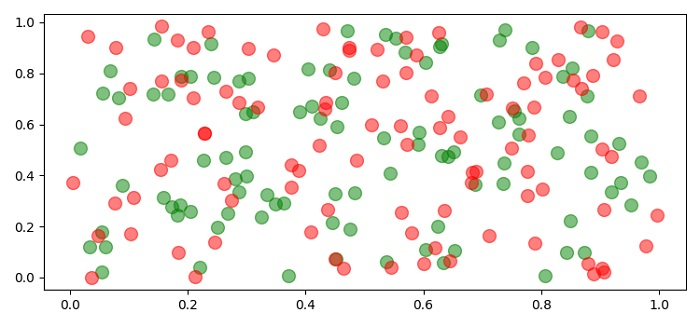# How to make Matplotlib scatterplots transparent as a group?

To make matplotlib scatterplots transparent as a group, we can change the alpha value in the scatter() method argument with a different group value.

## Steps

• Set the figure size and adjust the padding between and around the subplots.

• Make a method to return a grouped x and y points.

• Get group 1 and group 2 data points.

• Plot group1, x and y points using scatter() method with color=green and alpha=0.5.

• Plot group2, x and y points using scatter() method with color=red and alpha=0.5.

• To display the figure, use show() method.

## Example

import numpy as np
from matplotlib import pyplot as plt
plt.rcParams["figure.figsize"] = [7.50, 3.50]
plt.rcParams["figure.autolayout"] = True
def get_group_points():
return np.random.rand(100), np.random.rand(100),
group_1 = get_group_points()
group_2 = get_group_points()
plt.scatter(group_1, group_1, color='green', alpha=0.5, s=100)
plt.scatter(group_2, group_2, color='red', alpha=0.5, s=100)
plt.show()

## Output